## LeCun推荐：最新PyTorch图神经网络库，速度快15倍（GitHub+论文）

3 月 10 日 新智元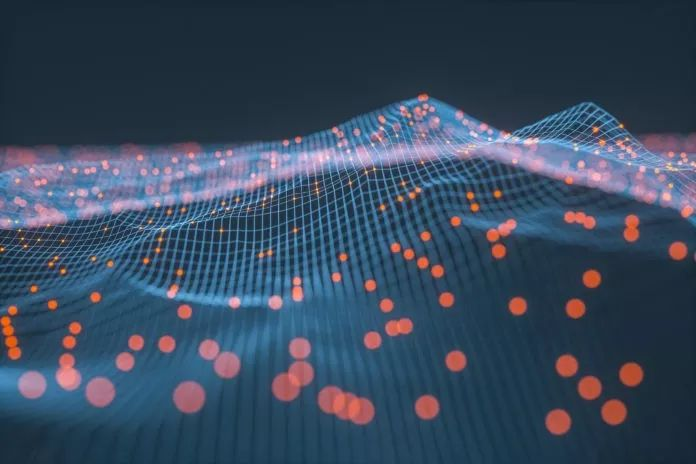新智元报道

##### 【新智元导读】德国研究者提出最新几何深度学习扩展库 PyTorch Geometric (PyG)，具有快速、易用的优势，使得实现图神经网络变得非常容易。作者开源了他们的方法，并提供教程和实例。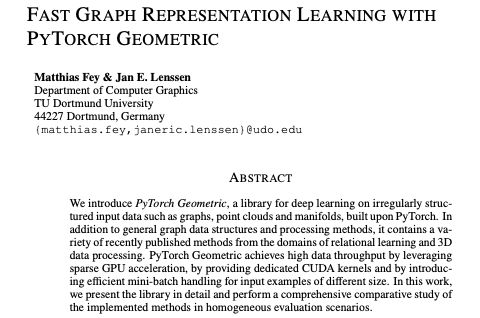https://arxiv.org/pdf/1903.02428.pdf

Yann Lecun 也热情推荐了这个工作，称赞它是一个快速、美观的 PyTorch 库，用于几何深度学习 (图和其他不规则结构的神经网络)。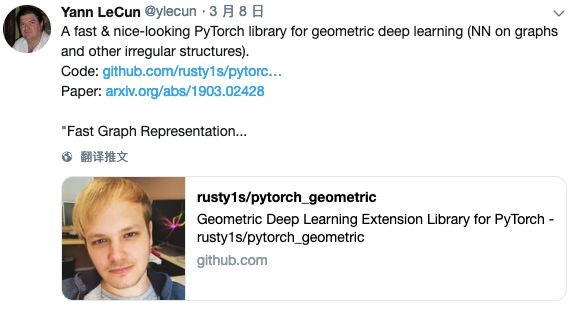PyG 已经在 MIT 许可下发布，可以在 GitHub 上获取。里面有完整的文档说明，并提供了作为起点的教程和示例。

https://github.com/rusty1s/pytorch_geometric

PyTorch Geometry：基于 PyTorch 的几何深度学习扩展库PyTorch Geometry 是一个基于 PyTorch 的几何深度学习扩展库，用于不规则结构输入数据，例如图 (graphs)、点云 (point clouds) 和流形 (manifolds)。

PyTorch Geometry 包含了各种针对图形和其他不规则结构的深度学习方法，也称为几何深度学习，来自于许多已发表的论文。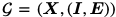Neighborhood Aggregation：将卷积算子推广到不规则域通常表示为一个邻域聚合(neighborhood aggregation)，或 message passing scheme (Gilmer et al., 2017)。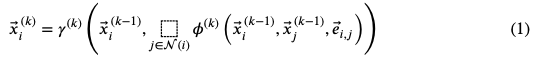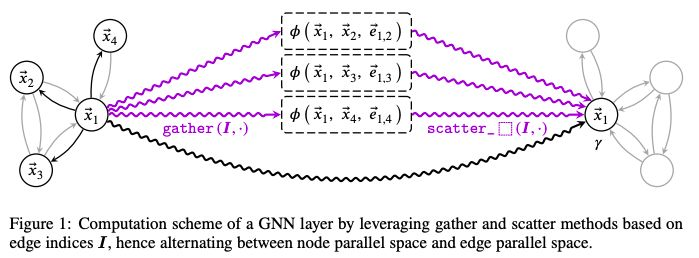• GCN (Kipf & Welling, 2017) 和它的简化版本 SGC (Wu et al., 2019)

• spectral chebyshev 和 ARMA filter convolutionss (Defferrard et al., 2016; Bianchi et al., 2019)

• GraphSAGE (Hamilton et al., 2017)

• attention-based operators GAT (Veličković et al., 2018) 及 AGNN (Thekumparampil et al., 2018),

• Graph Isomorphism Network (GIN) from Xu et al. (2019)

• Approximate Personalized Propagation of Neural Predictions (APPNP) operator (Klicpera et al., 2019)

• Schlichtkrull et al. (2018) 的 relational GCN operator

• PointNet++ (Qi et al., 2017)

• PointCNN (Li et al., 2018)

• kernel-based methods MPNN (Gilmer et al., 2017),

• MoNet (Monti et al., 2017)

• SplineCNN (Fey et al., 2018)

• 以及边缘卷积算子 EdgeCNN (Wang et al., 2018b).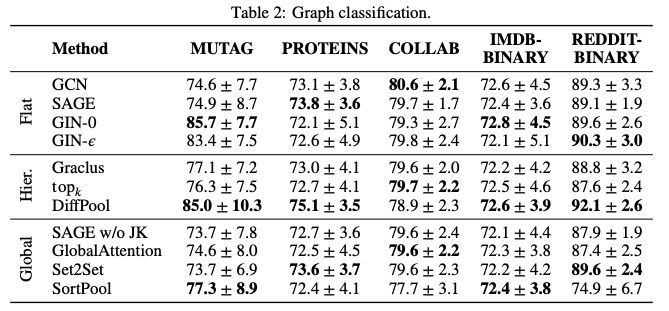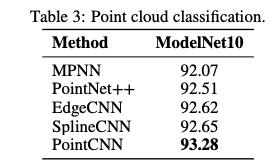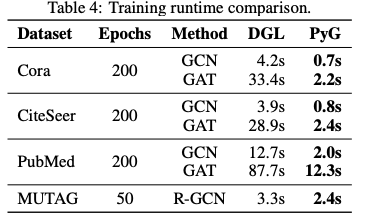PyTorch Geometric 使实现图卷积网络变得非常容易 (请参阅 GitHub 上的教程)。

``import torch``from torch.nn import Sequential as Seq, Linear as Lin, ReLU``from torch_geometric.nn import MessagePassing``class EdgeConv(MessagePassing):``    def __init__(self, F_in, F_out):``        super(EdgeConv, self).__init__()``        self.mlp = Seq(Lin(2 * F_in, F_out), ReLU(), Lin(F_out, F_out))``    def forward(self, x, edge_index):``        # x has shape [N, F_in]``        # edge_index has shape [2, E]``        return self.propagate(aggr='max', edge_index=edge_index, x=x)  # shape [N, F_out]``    def message(self, x_i, x_j):``        # x_i has shape [E, F_in]``        # x_j has shape [E, F_in]``        edge_features = torch.cat([x_i, x_j - x_i], dim=1)  # shape [E, 2 * F_in]``        return self.mlp(edge_features)  # shape [E, F_out]``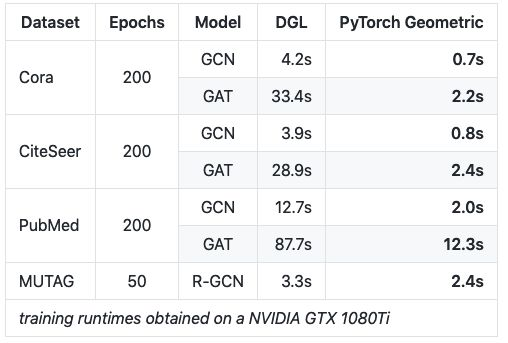``\$ python -c "import torch; print(torch.__version__)"``>>> 1.0.0``\$ echo \$PATH``>>> /usr/local/cuda/bin:...``\$ echo \$CPATH``>>> /usr/local/cuda/include:...``

``\$ pip install --upgrade torch-scatter``\$ pip install --upgrade torch-sparse``\$ pip install --upgrade torch-cluster``\$ pip install --upgrade torch-spline-conv (optional)``\$ pip install torch-geometric``

``cd examples``python cora.py``

paper：

https://arxiv.org/pdf/1903.02428.pdf

GitHub：

https://github.com/rusty1s/pytorch_geometric

• ## 1万7！华为震撼发布全球最快5G折叠屏手机，余承东炫业界最大创新

【加入社群】0+12+

### 更多0+5+0+0+18+0+0+3+0+0+4+0+0+9+0+0+4+0+0+5+0+0+4+0+0+11+0+0+4+0+
Top